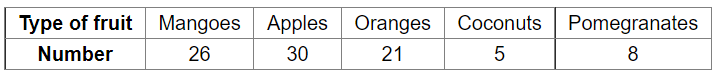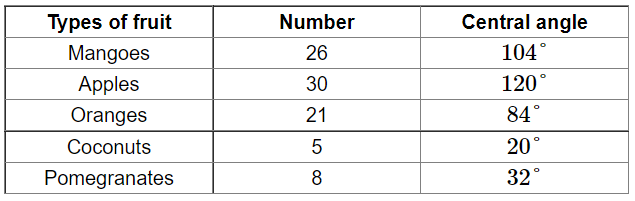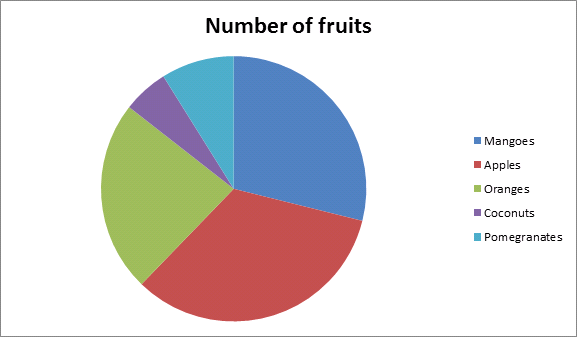# The following table gives the number of different fruits kept in a hamper.

Question:

The following table gives the number of different fruits kept in a hamper.Represent the above data by a pie chart.

Solution:

Total number of fruits $=(26+30+21+5+8)=90$

Central angle of each fruit $=\left(\frac{\text { number of each type of fruit }}{\text { total number of fruits }} \times 360\right) \circ$

Calculation of central anglesConstruction of pie chart

Steps of construction:

1. Draw a circle of any convenient radius.

2. Draw a horizontal radius of the circle.

3. Draw sectors whose central angles are $104^{\circ}, 120^{\circ}, 84^{\circ}, 20^{\circ}$ and $32^{\circ} .$

4. Shade the sectors so obtained differently and label each one of them.

Thus, we obtain the required pie chart as shown in the figure below.